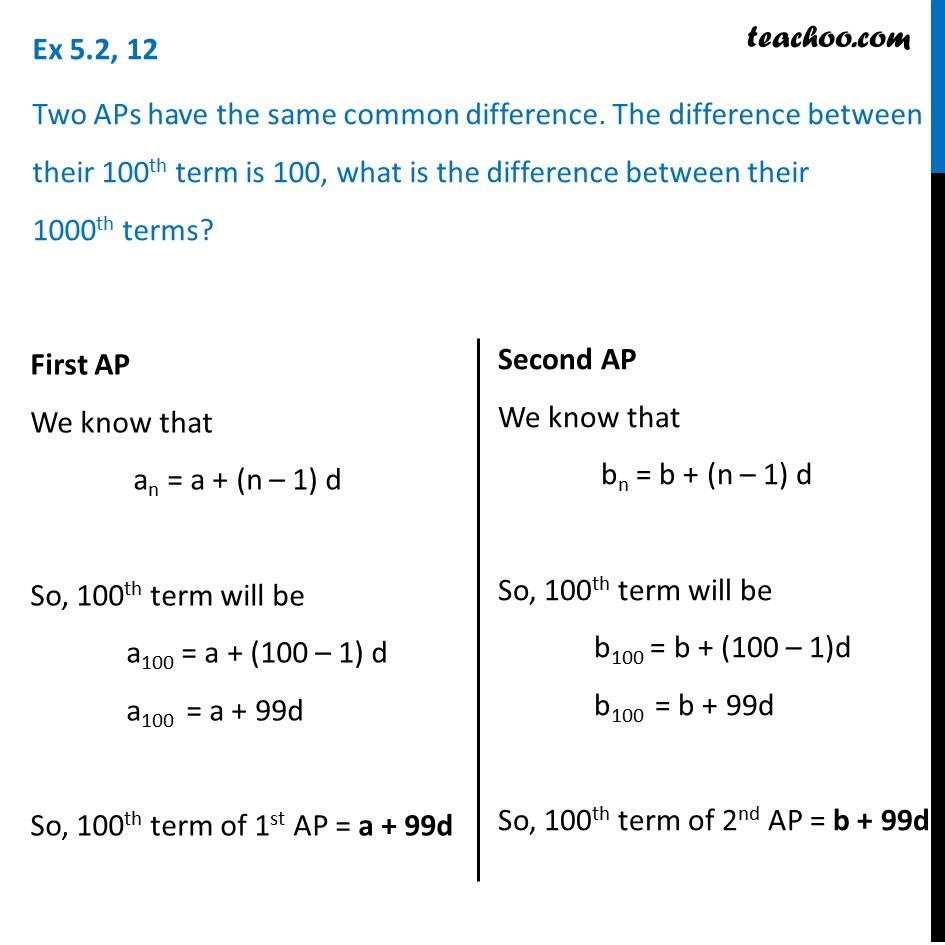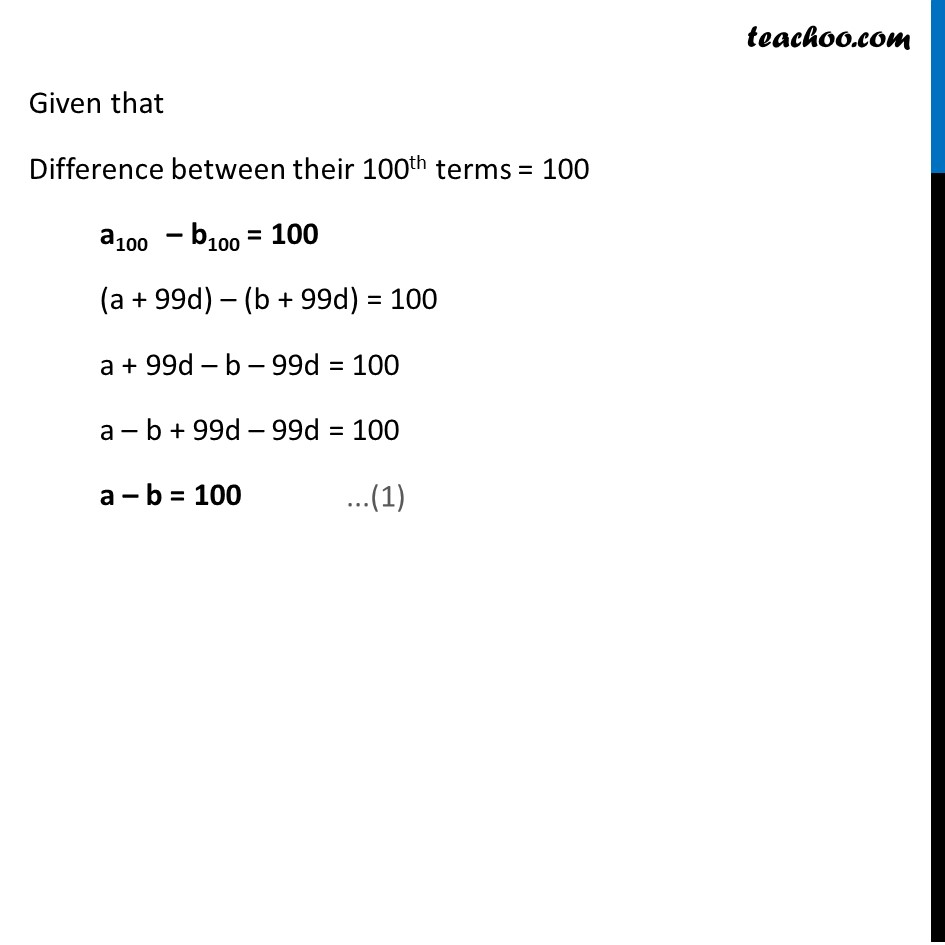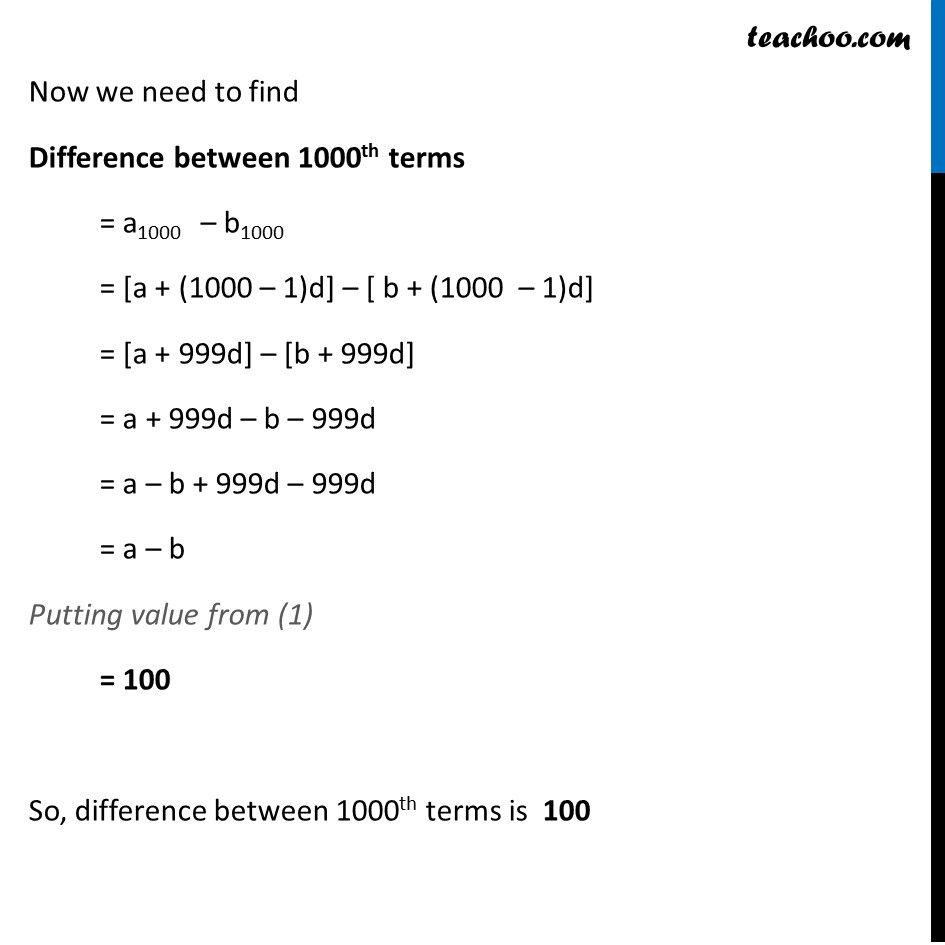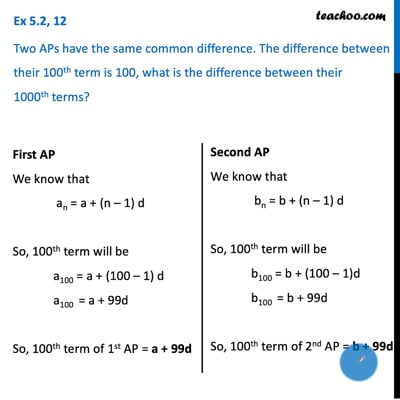Finding AP

Chapter 5 Class 10 Arithmetic Progressions (Term 2)
Concept wiseThis video is only available for Teachoo black users

### Transcript

Ex 5.2, 12 Two APs have the same common difference. The difference between their 100th term is 100, what is the difference between their 1000th terms? First AP We know that an = a + (n – 1) d So, 100th term will be a100 = a + (100 – 1) d a100 = a + 99d So, 100th term of 1st AP = a + 99d Second AP We know that bn = b + (n – 1) d So, 100th term will be b100 = b + (100 – 1)d b100 = b + 99d So, 100th term of 2nd AP = b + 99d Given that Difference between their 100th terms = 100 a100 – b100 = 100 (a + 99d) – (b + 99d) = 100 a + 99d – b – 99d = 100 a – b + 99d – 99d = 100 a – b = 100 ...(1) Now we need to find Difference between 1000th terms = a1000 – b1000 = [a + (1000 – 1)d] – [ b + (1000 – 1)d] = [a + 999d] – [b + 999d] = a + 999d – b – 999d = a – b + 999d – 999d = a – b Putting value from (1) = 100 So, difference between 1000th terms is 100